# K-Means Clustering From Scratch Python – Free Machine Learning Course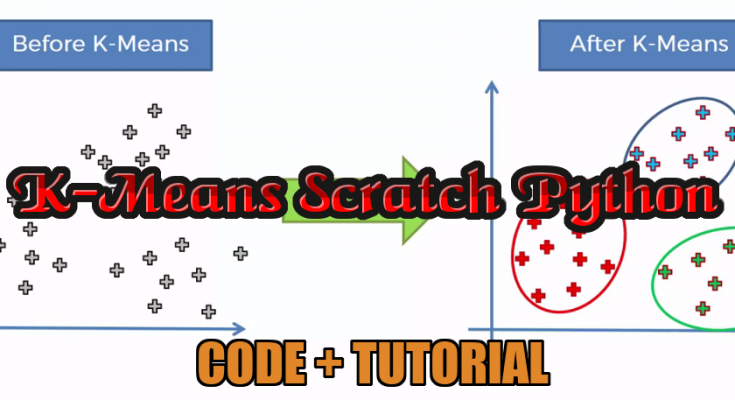In this article, we will cover k-means clustering from scratch. In general, Clustering is defined as the grouping of data points such that the data points in a group will be similar or related to one another and different from the data points in another group. The goal of clustering is to determine the intrinsic grouping in a set of unlabelled data.

K- means is an unsupervised partitional clustering algorithm that is based on grouping data into k – numbers of clusters by determining centroid using the Euclidean or Manhattan method for distance calculation. It groups the object based on minimum distance.

#### ALGORITHM

• 1. First,  initialize the number of clusters, K (Elbow method is generally used in selecting the number of clusters )
• 2. Randomly select the k data points for centroid. A centroid is the imaginary or real location representing the center of the cluster.
• 3. Categorize each data items to its closest centroid and update the centroid coordinates calculating the average of items coordinates categorized in that group so far
• 4. Repeat the process for a number of iterations till successive iterations clusters data items into the same group

#### HOW IT WORKS ?

In the beginning, the algorithm chooses k centroids in the dataset randomly after shuffling the data. Then it calculates the distance of each point to each centroid using the euclidean distance calculation method. Each centroid assigned represents a cluster and the points are assigned to the closest cluster. At the end of the first iteration, the centroid values are recalculated, usually taking the arithmetic mean of all points in the cluster.  In every iteration, new centroid values are calculated until successive iterations provide the same centroid value.

Let’s kick off K-Means Clustering Scratch with a simple example: Suppose we have data points (1,1), (1.5,2), (3,4), (5,7), (3.5,5), (4.5,5), (3.5,4.5). Let us suppose k = 2 i.e. dataset should be grouped in two clusters. Here we are using the Euclidean distance method.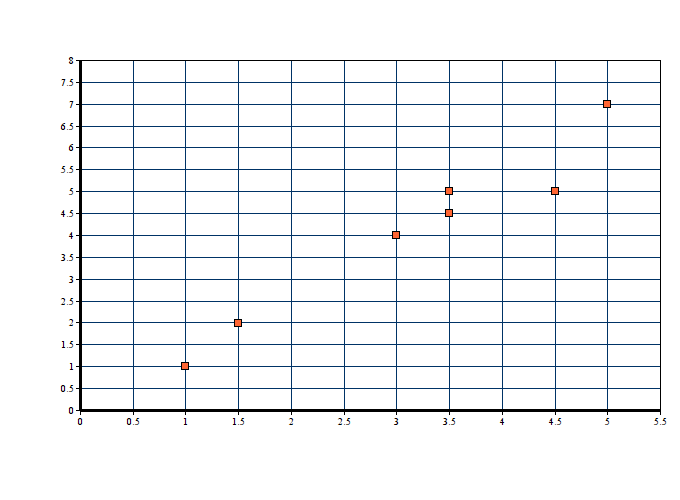Step 1 : It is already defined that k = 2 for this problem

Step-2:  Since k = 2, we are randomly selecting two centroid as c1(1,1) and c2(5,7)

Step 3: Now, we calculate the distance of each point to each centroid using the euclidean distance calculation method: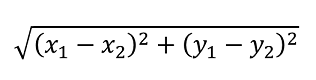##### ITERATION 01

Note:  D1 & D2 are euclidean distance between centroid (x2,y2) and data points (x1,y1)

In cluster c1 we have (1,1), (1.5,2) and (3,4) whereas centroid c2 contains (5,7), (3.5,5), (4.5,5) &  (3.5,4.5). Here, a new centroid is the algebraic mean of all the data items in a cluster.

• C1(new) = ( (1+1.5+3)/3 , (1+2+4)/3) = (1.83, 2.33)
• C2(new) = ((5+3.5+4.5+3.5)/4, (7+5+5+4.5)/4) = (4.125, 5.375)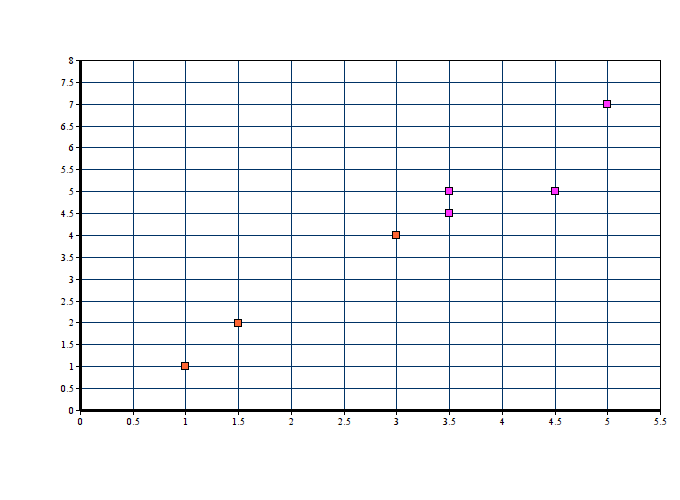##### ITERATION 02

In cluster c1 we have (1,1), (1.5,2) ) whereas centroid c2 contains (3,4),(5,7), (3.5,5), (4.5,5) &  (3.5,4.5). Here, new centroid is the algebraic mean of all the data items in a cluster.

• C1(new) = ( (1+1.5)/2 , (1+2)/2) = (1.25,1.5)
• C2(new) = ((3+5+3.5+4.5+3.5)/5, (4+7+5+5+4.5)/5) = (3.9, 5.1)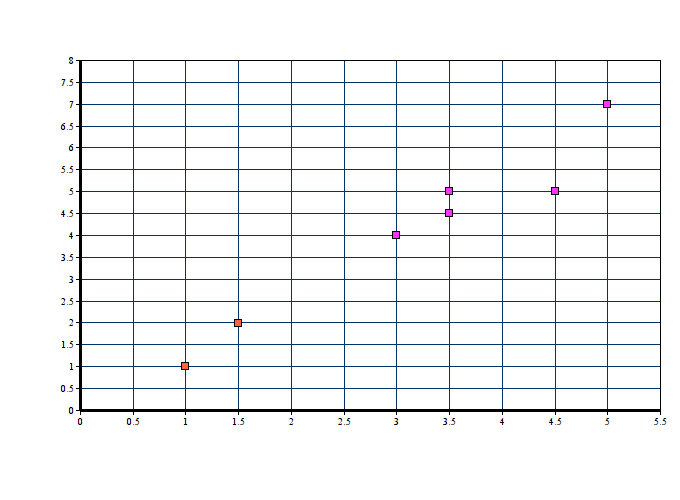##### ITERATION 03

In cluster c1 we have (1,1), (1.5,2) ) whereas centroid c2 contains (3,4),(5,7), (3.5,5), (4.5,5) &  (3.5,4.5). Here, new centroid is the algebraic mean of all the data items in a cluster.

• C1(new) = ( (1+1.5)/2 , (1+2)/2) = (1.25,1.5)
• C2(new) = ((3+5+3.5+4.5+3.5)/5, (4+7+5+5+4.5)/5) = (3.9, 5.1)

Step 04: In the 2nd and 3rd iteration, we obtained the same centroid points. Hence clusters of above data point is :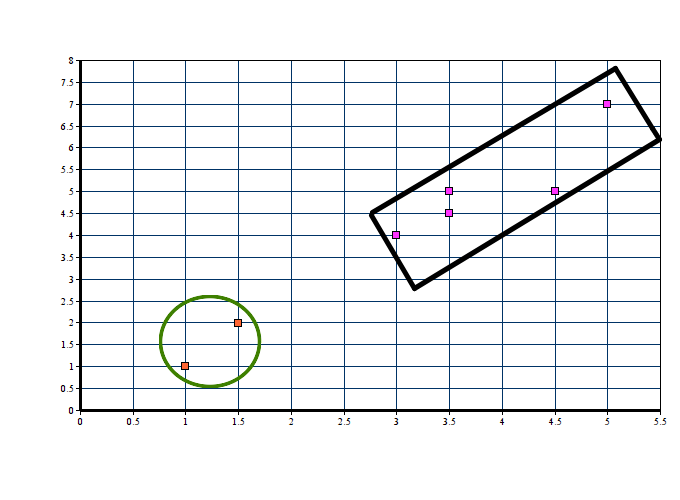## K-Means Clustering Scratch Code

So far, we have learnt about the introduction to the K-Means algorithm. We have learnt in detail about the mathematics behind the K-means clustering algorithm and have learnt how Euclidean distance method is used in grouping the data items in K number of clusters. Here were are implementing K-means clustering from scratch using python. But the problem is how to choose the number of clusters? In this example, we are assigning the number of clusters ourselves and later we will be discussing various ways of finding the best number of clusters. This code is referenced from Muthu.

We have defined a K-means class with init consisting default value of k as 2, error tolerance as 0.001, and maximum iteration as 500.
Before diving into the code, let’s remember some mathematical terms involved in K-means clustering:- centroids & euclidean distance. On a quick note centroid of a data is the average or mean of the data and Euclidean distance is the distance between two points in the coordinate plane calculated using Pythagoras theorem.

We find the euclidean distance from each point to all the centroids. If you look for efficiency it is better to use the NumPy function (np.linalg.norm(point1-point2, axis=0))

##### ASSIGNING CENTROIDS

There are various methods of assigning k centroid initially. Mostly used is a random selection but let’s go in the most basic way. We assign the first k points from the dataset as the initial centroids.

Till now, we have defined the K-means class and initialized some default parameters. We have defined the euclidean distance calculation function and we have also assigned initial k clusters. Now, In order to know which cluster and data item belong to, we are calculating Euclidean distance from the data items to each centroid. Data item closest to the cluster belongs to that respective cluster.

At the end of the first iteration, the centroid values are recalculated, usually taking the arithmetic mean of all points in the cluster. In every iteration, new centroid values are calculated until successive iterations provide the same centroid value.

##### CLUSTERING WITH DEMO DATA

We’ve now completed the K Means scratch code of this Machine Learning tutorial series. Now, let’s test our code by clustering with randomly generated data:

Here we have created 3 groups of data of two-dimension with a different centre. We have defined the value of k as 3. Now, let’s fit the model created from scratch.

#### CHOOSING VALUE OF K

While working with the k-means clustering scratch, one thing we must keep in mind is the number of clusters ‘k’. We should make sure that we are choosing the optimum number of clusters for the given data set.  But, here arises a question, how to choose the optimum value of k ?? We use the elbow method which is generally used in analyzing the optimum value of k.

The Elbow method is based on the principle that “Sum of squares of distances of every data point from its corresponding cluster centroid should be as minimum as possible”.

#### STEPS OF CHOOSING BEST K VALUE

• 1. Run k-means clustering model on various values of k
• 2. For each value of K, calculate the Sum of squares of distances of every data point from its corresponding cluster centroid which is called WCSS ( Within-Cluster Sums of Squares)
• 3. Plot the value of WCSS with respect to various values of K
• 4. To select the value of k, we choose the value where there is bend (knee) on the plot i.e. WCSS isn’t increasing rapidly.

#### PROS OF K-MEANS

• 1. Relatively simple to learn and understand as the algorithm solely depends on the euclidean method of distance calculation.
• 2. K means works on minimizing Sum of squares of distances, hence it guarantees convergence
• 3. Computational cost is O(K*n*d), hence K means is fast and efficient

#### CONS OF K-MEANS

• 1. Difficulty in choosing the optimum number of clusters K
• 2. K means has a problem when clusters are of different size, densities, and non-globular shapes
• 3. K means has problems when data contains outliers
• 4. As the number of dimensions increases, the difficulty in getting the algorithm to converge increases due to the curse of dimensionality
• 5. If there is overlapping between clusters, k-means doesn’t have an intrinsic measure for uncertainty
##### Get Project Codes FREE
View All### Hand Detection & Gesture Recognition – OpenCV Python

Hand gesture recognition is one of the most viable and popular solution for improving human computer interaction. In the recent years it has become very popular due to its use …

### Chrome Dinosaur Game using Python – Free Code Available